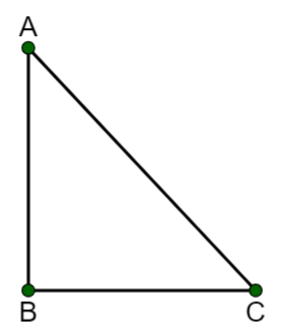Edumarz# Motion

Description

Tanusri Gururaj, Academic content writer of Physics at Edumarz

The motion of any object is described using the following terms-

• Distance: It is the length of the path covered by an object. It is a scalar quantity and hence, it has magnitude but no direction.

• Displacement: It is the shortest distance between the initial and final points. It has both magnitude and direction since it is a vector quantity.

• Speed: It is defined as the distance that an object travels per unit of time.

• Velocity: It is the distance travelled in a given direction per unit of time.

• Acceleration: The measure of how fast the velocity of an object changes.

• Time: The duration of any particular event.

Understanding distance and displacement with an example-Consider this example of a triangular path.

Let us assume that a person goes from path A to B and then from B to C.

The distance is the length of the actual path covered by the person that is AB + BC.

The displacement is the shortest distance between the initial and final points and hence will be AC.

Understanding speed and velocity with an example-

52 km/hr is the speed of a truck ‘A’ on a highway, whereas 52 km/hr east is the velocity of a truck ‘B.’

The explanation behind this is that in the case of truck ‘B,’ 52 km/hr east gives both magnitude and direction, where 52 km/hr is the magnitude and east is the direction.

Formulae related to this concept-

1. Speed = distance/time

Unit = m/s

1. Velocity = displacement/time

Unit = m/s

1. Acceleration = Change in velocity of the object/time taken for the velocity change

Change in velocity = final velocity of object – initial velocity of object

Unit = m/s2

Uniform Motion

The object undergoing uniform motion covers equal distances in equal intervals of time.

For example, a car covers 20m in the first second, the next 20m in the next second, and so on.

Non-uniform motion

In this type of motion, the particular object travels unequal distances in equal intervals of time.

For example, a car covers 1km in the first 35 seconds, 0.9km in the next 35 seconds.## AMU, a top-notch opportunity## AIMS Test for Management## BANARAS HINDU UNIVERSITY